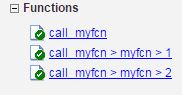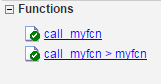coder.ignoreConst

Prevent use of constant value of expression for function specializations

Description

example

coder.ignoreConst(expression) prevents the code generator from using the constant value of expression to create function specializations. coder.ignoreConst(expression) returns the value of expression.

Examples

collapse all

Use coder.ignoreConst to prevent function specializations for a function that is called with constant values.

Write the function call_myfn, which calls myfcn.

function [x, y] = call_myfcn(n)
%#codegen
x = myfcn(n, 'mode1');
y = myfcn(n, 'mode2');
end

function y = myfcn(n,mode)
coder.inline('never');
if strcmp(mode,'mode1')
y = n;
else
y = -n;
end
end

Generate standalone C code. For example, generate a static library. Enable the code generation report.

codegen -config:lib call_myfcn -args {1} -report

In the code generation report, you see two function specializations for call_myfcn.The code generator creates call_myfcn>myfcn>1 for mode with a value of 'mode1'. It creates call_myfcn>myfcn>2 for mode with a value of 'mode2'.

In the generated C code, you see the specializations my_fcn and b_my_fcn.

static double b_myfcn(double n)
{
return -n;
}

static double myfcn(double n)
{
return n;
}

To prevent the function specializations, instruct the code generator to ignore that values of the mode argument are constant.

function [x, y] = call_myfcn(n)
%#codegen
x = myfcn(n, coder.ignoreConst('mode1'));
y = myfcn(n, coder.ignoreConst('mode2'));
end

function y = myfcn(n,mode)
coder.inline('never');
if strcmp(mode,'mode1')
y = n;
else
y = -n;
end
end

Generate the C code.

codegen -config:lib call_myfcn -args {1} -report

In the code generation report, you do not see multiple function specializations.In the generated C code, you see one function for my_fcn.

Input Arguments

collapse all

Expression whose value is to be treated as a nonconstant, specified as a MATLAB expression.

collapse all

Function Specialization

Version of a function in which an input type, size, complexity, or value is customized for a particular invocation of the function.

Function specialization produces efficient C code at the expense of code duplication. The code generation report shows all MATLAB function specializations that the code generator creates. However, the specializations might not appear in the generated C/C++ code due to later transformations or optimizations.

Tips

• For some recursive function calls, you can use coder.ignoreConst to force run-time recursion. See Force Code Generator to Use Run-Time Recursion.

• coder.ignoreConst(expression) prevents the code generator from using the constant value of expression to create function specializations. It does not prevent other uses of the constant value during code generation.

Extended Capabilities

GPU Code GenerationGenerate CUDA® code for NVIDIA® GPUs using GPU Coder™.

Introduced in R2017a